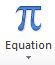# Tips, Tricks & Other Helpful Hints: Equation editorNeed to format an equation in a Word document? Here's how!

There can be many instances in which equations need to be included in a document to accurately express information. When you type an equation, Word can convert the equation into a professionally formatted equation.

1. On the "Insert" tab, in the "Symbols" group, click the arrow under "Equation."

2. Click "Insert New Equation" to type an equation.

3. Use the symbols and structures provide on the "Equation Editor Design" tab to create the necessary equation.

NOTE: The equation often may be centered on the line in which it appears. It can be changed to any sort of alignment.

4. For editing equation values you need to click inside equation box to change values manually.

Many of these features of Equation Editor can be used in other Microsoft programs.

These instructions, along with illustrations and instructions for additional features of Equation Editor, can also be found in SharePoint > Software Users Group > Shared Documents > Word > Equation Editor.